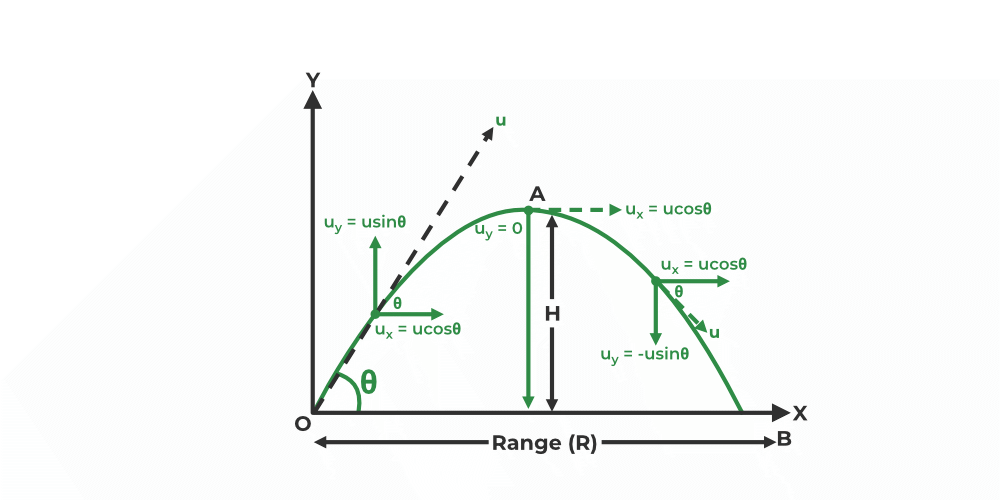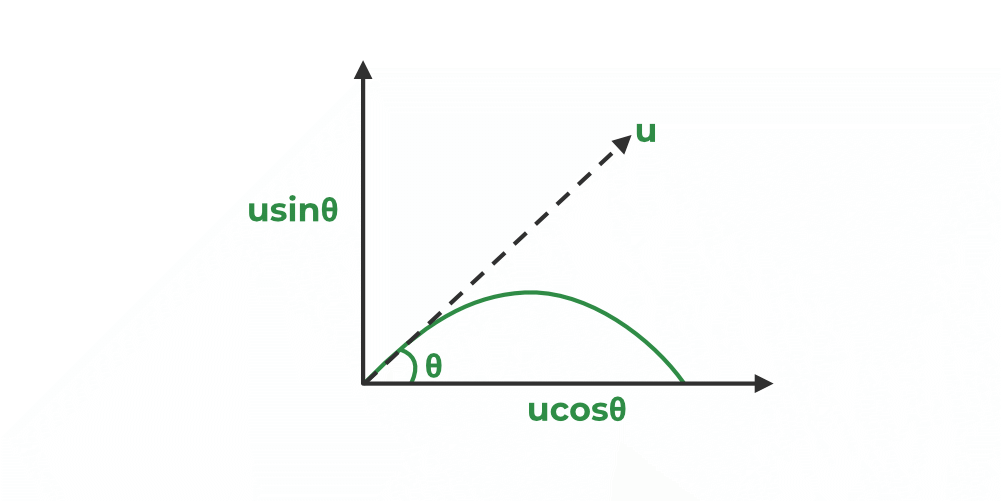Open in App
Not now

# Derivation of Equation of Trajectory of a Projectile Motion

• Last Updated : 08 Jan, 2023

An object that is launched into space with only gravity acting on it is known as a projectile. The main force that acts on a projectile is gravity. Though other forces like air resistance also act on a projectile, their impact on the projectile is minimal when compared to the gravitational force. An arrow released from a bow, the launching of missiles, a bullet fired from a gun, a javelin thrown by an athlete, a ball thrown into the air, etc. are some real-life examples of projectiles.

## Trajectory of a Projectile Motion

An object that is thrown into the air moves along a curved path under constant acceleration that is pointed toward the center of the earth. The object that is propelled into space is referred to as a projectile, and the trajectory is the path traveled by the projectile. When the object is launched into space, some initial force must be imparted upon it such that it becomes a projectile. Projectile motion is defined as the motion of a body in flight after being propelled into space.In a projectile motion, there are two components of motion, i.e., motion along the horizontal direction without any acceleration and motion along the vertical direction under the influence of constant acceleration due to the force of gravity. An object that is thrown into space with an initial velocity and at an angle (except the right angle) with respect to the horizontal travels in a curved path under the influence of acceleration due to the force of gravity. The path of a projectile during its flight is predictable, i.e., a parabolic shape in the absence of air resistance.

## Derivation of Equation of Trajectory

The equation of trajectory will give the relation between the x and y coordinates at any point in the flight of a projectile and is also used to determine the path of the projectile. Consider that an object is launched into space with an initial velocity of “u” and at an angle “θ” with respect to the horizontal.The horizontal component of the initial velocity ux = u cosθ and the vertical component of the initial velocity uy = u sinθ.

There is no displacement along the X and Y axes when t = 0 sec.

When T = t,

As the acceleration due to the force of gravity doesn’t act on the projectile in the horizontal direction, the velocity of the projectile will not change in this direction.

So, vx = ux

The horizontal displacement of a projectile (x) = uxt = (u cosθ) t… (1)

As the acceleration due to the force of gravity acts vertically down, the velocity of the projectile changes in the vertical direction.

vy = (u sinθ)t − gt

The vertical displacement of a projectile (y) = uyt − ½gt2 … (2)

From equation (1), t = x/ (u cosθ)… (3)

Now, substitute the value of “t” in equation (2)

y = (u sinθ) × x/ (u cosθ) − [½ × g × (x/ (u cosθ))2]

y = x tanθ − [gx2/2(ucosθ)2

y = x tanθ − [gx2/2u2cos2θ] … (4)

In the above equation, the angle of projection (θ), acceleration due to gravity (g), and the initial velocity (u) are all constants.

So, by rewriting equation (4), we get

y = ax + bx2, where “a” and “b” are constants.

We can observe that this equation represents a parabola. So, we can conclude that the path of a projectile during its flight is parabolic.

## Trajectory Formula

The formula explaining the path of projectile motion is discussed below, for other trajectory formulas click here.where

• “y” is the vertical distance of the object,
• “x” is the horizontal distance of the object,
• “u” is the initial velocity of the object,
• “θ” is the angle of projection with respect to the horizontal,
• “g” is the acceleration due to gravity.

Also, Check

## Solved Examples on Projectile Motion

Example 1: Determine the vertical distance of the ball that is launched from some distance, making the projectile motion. The ball was thrown with an initial velocity of 40 m/s at an angle of 45°, and it hit the ground after 4 seconds. (g = 10 m/s2)

Solution:

Given data:

Angle (θ) = 45°

Time = 4 sec

Initial Velocity (u) = 40 m/s

Horizontal distance (x) = ux

x = ucosθ t

x = 40 × cos 45° × 4 = 113.137 m

From the trajectory formula, we have,

y = x tanθ − [gx2/2u2cos2θ]

y = 113.137 × tan 45° − [10 × (113.137)2/2 × (40)2 × (cos 45°)2]

y = 113.137 × 1 − [10 × 12,799.981/2 × 1600 × (1/√2)2]

y = 113.137 − [1,27,999.81/1600]

y = 113.137 − 79.99

= 33.147 m

Hence, the vertical distance covered by the ball is 33.147 m.

Example 2: A bullet is fired from a gun with a velocity of 15 m/s at an angle of 60°. Find the equation for the path of a projectile using the trajectory formula. (g = 9.8 m/s2)

Solution:

Given data:

Initial Velocity (u) = 15 m/s

Angle (θ) = 60°

From the trajectory formula, we have,

y = x tanθ − [gx2/2(ucosθ)2]

y = x tan 60° − [9.8 × x2/2(15 × cos 60°)2]

y = x × (√3) − [9.8x2/2 × (225/4)]

y = √3x – 0.087x2

Thus, the equation of the trajectory of the projectile is y =√3x – 0.087x2.

Example 3: Determine the vertical distance of the ball that is launched from some distance, making the projectile motion. The ball was thrown with an initial velocity of 13 m/s at an angle of 30°, and it hit the ground after 5 seconds. (g = 9.8 m/s2)

Solution:

Given data:

Angle (θ) = 30°

Time = 5 sec

Initial Velocity (u) = 13 m/s

Horizontal distance (x) = uxt

x = ucosθ t

x = 13 × cos 30° × 5 = 56.29 m

From the trajectory formula, we have,

y = x tanθ − [gx2/2u2cos2θ]

y = 56.29 × tan 30° − [9.8 × (56.29)2/2 × (13)2 × (cos 30°)2]

y = 56.29 × 1/√3 − [9.8 × 3,168.5641/2 × 169 × (√3/2)2]

y = 32.49 − [31,051.928/253.5]

y = 32.49 − 122.49

= −89.99 m

Example 4: A boy threw a stone with a velocity of 9 m/s at an angle of 35°. Find the equation for the path of a projectile using the trajectory formula. (g = 10 m/s2)

Solution:

Given data:

Initial Velocity (u) = 9 m/s

Angle (θ) = 35°

From the trajectory formula, we have,

y = x tanθ − [gx2/2(ucosθ)2]

y = x tan 35° − [10 × x2/2(9 × cos 35°)2]

y = x × (0.7002) − [10x2/2 × (9 × 0.819)2]

y = (0.7002) x – (0.0920) x2

Thus, the equation of the trajectory of the projectile is y = (0.7002) x – (0.0920) x2.

## FAQs on Projectile Motion

### Question 1: What is meant by a projectile? Give some examples of the projectile.

An object that is launched into space with only gravity acting on it is known as a projectile. An arrow released from a bow, the launching of missiles, a bullet fired from a gun, a javelin thrown by an athlete, a ball thrown into the air, etc. are some real-life examples of projectiles.

### Question 2: What is meant by a trajectory?

The object that is propelled into space is referred to as a projectile, and the trajectory is the path traveled by the projectile.

### Question 3: What is the trajectory formula?where

• “y” is the vertical distance of the object,
• “x” is the horizontal distance of the object,
• “u” is the initial velocity of the object,
• “θ” is the angle of projection with respect to the horizontal, and
• “g” is the acceleration due to gravity.